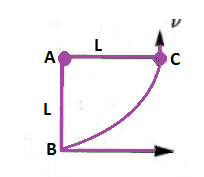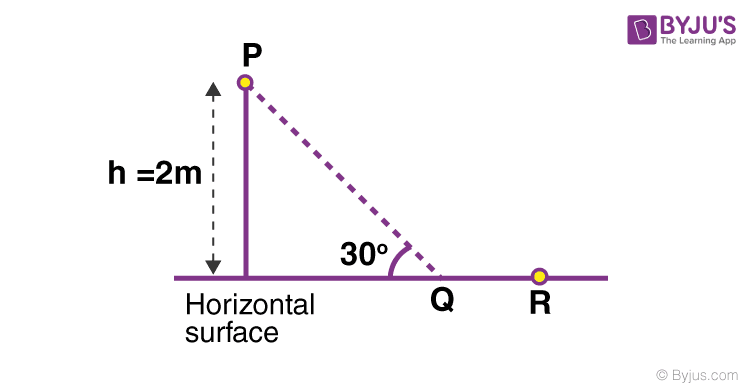# JEE Main Work, Power and Energy Previous Year Questions with Solutions

Work is done when there is a motion of the object with the application of external force. Work is calculated as the product of force and the displacement of the object. For example, a force of 20 newtons (N) pushing an object 5 meters in the same direction of the force will do 100 joules (J) of work.

Energy, in simple words, is defined as the ability to do work. Energy is transformed from one form to another and it cannot be destroyed. Energy is in different forms like mechanical energy, electrical energy, Kinetic energy, potential energy and many more.

Power is generally defined as the rate of doing work. It is the amount of energy consumed per unit time.

Work, Power and Energy is an important chapter in JEE. Solving previous years’ question papers helps the students to analyse the pattern of questions and to understand the method of studies.

## JEE Main Previous Year Solved Questions on Work, Power and Energy

Q1: Two masses of 1 g and 4g are moving with equal kinetic energy. The ratio of the magnitudes of their momenta is

(a) 4 : 1

(b) $\sqrt{2}:1$

(c) 1: 2

(d) 1 : 16

Solution: $p=\sqrt{2Em}$

Where p denotes momentum, E denotes kinetic energy and mdenotes mass.

$\frac{p_{1}}{p_{2}}=\sqrt{\frac{m_{1}}{m_{2}}}$ $\frac{p_{1}}{p_{2}}=\sqrt{\frac{1}{4}}=\frac{1}{2}$

Q2: If a machine is lubricated with oil

(a) the mechanical advantage of the machine increases

(b) the mechanical efficiency of the machine increases

(c) both its mechanical advantage and efficiency increase

(d) its efficiency increases, but its mechanical advantage decreases.

Answer.(b) When a machine is lubricated with oil friction decreases. Hence the mechanical efficiency of the machine increases.

Q3: The potential energy function for the force between two atoms in a diatomic molecule is approximately given by $U(x)=\frac{a}{x^{12}}-\frac{b}{x^{6}}$, where a and b are constant and x is the distance between the atoms. if the dissociation energy of the molecule is (U(x= ∞) -U at equilibrium), D is

(a) b2/6a

(b) b2/2a

(c) b2/12a

(d) b2/4a

Solution

U(x= ∞) = 0,at equilibrium

dU/dx = 0

$U(x)=\frac{a}{x^{12}}-\frac{b}{x^{6}}$——-(1)

-12ax-13 + 6bx-7 = 0

-12a/x13 = 6b/x7

x6 = 2a/b———-(2)

From 1 and 2 we have

$U=\frac{a-b(2a/b)}{(2a/b)^{2}}$

U = -b2/4a

D = (0 + b2/4a)

D = b2/4a

Q4: At time t = 0s particle starts moving along the x-axis. If its kinetic energy increases uniformly with time ‘t’, the net force acting on it must be proportional to

(a) $\sqrt{t}$

(b) constant

(c) t

(d) $\frac{1}{\sqrt{t}}$

Solution

Linear dependency with initial Kinetic Energy as zero is given as KE = kt, where k is the proportionality constant.

Kinetic energy can be written as KE= ½ mv2 so we can write

½ mv2 = kt

$v = \sqrt{\frac{2kt}{m}}$ $\frac{dv}{dt} = \sqrt{\frac{k}{2mt}}$

F = m.dv/dt

$F = m\sqrt{\frac{k}{2mt}}$

F∞1/ $\sqrt{t}$

Answer:(d) 1/ $\sqrt{t}$

Q5: This question has Statement-1 and Statement-2. Of the four choices given after the statements, choose the one that best describes the two statements.

If two springs S1 and S2 of force constants k1 and k2, respectively, are stretched by the same force, it is found that more work is done on spring S1 than on spring S2.

Statement-1: If stretched by the same amount, work done on S1, will be more than that on S2

Statement-2 :k1 < k2

(a) Statement-1 is True, Statement-2 is true and Statement-2 is not the correct explanation of Statement-1.

(b) Statement-1 is False, Statement-2 is true

(c) Statement-1 is True, Statement-2 is false

(d) Statement-1 is True, Statement-2 is true and Statement-2 is the correct explanation of statement-1.

Solution

Given same force F = k1x1 = k2x2

k1/k2 = x1/x2

W1 = ½ k1x12 and W2=½ k2x22

As W1/W2 ＞1 so ½ k1x12/ ½ k2x22＞1

Fx1/Fx2＞1 ⇒ k2//k1＞1

Therefore k2 ＞ k1 Statement-2 is true

Or if x1 = x2 = x

W1/W2 = ½ k1x2 / ½ k2x2

W1/W2 = k1/ k2 ＜1

W1 ＜W2 , Statement-1 is False

Answer: (b) Statement-1 is False, Statement-2 is true

Q6: A particle of mass m is moving in a circular path of constant radius r such that its centripetal acceleration ac is varying with time ‘t’ as ac = k2rt2 where ‘k’ is a constant. The power delivered to the particle by the force acting on it is

(a) 2πmk2r2t

(b) mk2r2t

(c) mk4r2t5/3

(d) zero

Solution: Centripetal acceleration ac= k2rt2

v2/r= k2rt2

v2= k2r2t2

mv2/2=m k2r2t2/2

Kinetic energy = m k2r2t2/2

$\frac{d}{dt}(K.E) = mk^{2}r^{2}t$

Power = m k2r2t

Q7: A stone tied to a string of length L is whirled in a vertical circle with the other end of the string at the centre. At a certain instant of time, the stone is at its lowest position, and has a speed u. The magnitude of the change in its velocity as it reaches a position where the string is horizontal is

(a) $\sqrt{u^{2}-2gL}$

(b) $\sqrt{u^{2}-gL}$

(c) $\sqrt{2(u^{2}-gL)}$

(d) $\sqrt{2gL}$

Solution:At lowest position B, Potential Energy = 0

Kinetic Energy = ½ mu2

Total Energy = ½ mu2

At C, when string is horizontal,

Potential Energy = mgL

Kinetic Energy = ½ mv2

Total Energy = ½ mv2 + mgL

Since energy is conserved,

½ mv2 + mgL = ½ mu2

v2 = u2 -2gL

Since v is in vertical direction and u is in horizontal direction, they are mutually perpendicular to each other.

Change in velocity = $\sqrt{u^{2}+v^{2}}$ $\left | \Delta \vec{v} \right |=\sqrt{u^{2}+(u^{2}-2gL)}$ $\left | \Delta \vec{v} \right |=\sqrt{2(u^{2}-gL))}$

Answer: (c) $\sqrt{2(u^{2}-gL)}$

Q8: When a rubber-band is stretched by a distance x, it exerts a restoring force of magnitude F =ax + bx2 where a and b are constants. The work done in stretching the unstretched rubber-band by L is

(a) aL2/2 +bL3/3

(b) ½ (aL2/2 +bL3/3)

(c ) aL2 +bL3

(d) ½ (aL2 +bL3)

Solution

Work done by a variable force

$W = \int \vec{F}.\vec{ds}$

Wherein $\vec{F}$ is the variable force and $\vec{ds}$ is small displacement

F = ax + bx2

Work done in displacing rubber through dx = Fdx

$W = \int_{0}^{L}(ax + bx^{2})dx$

W = aL2/2 +bL3/3

Q9: A person trying to lose weight by burning fat lifts a mass of 10 kg up to a height of 1 m 1000 times. Assume that the potential energy lost each time he lowers the mass is dissipated. How much fat will he use up considering the work done only when the weight is lifted up? Fat supplies 3.8 X 107 J of energy per kg which is converted to mechanical energy with a 20% efficiency rate. Take g = 9.8 m/s2

(a) 12.89 X 10-3kg

(b) 2.45 X 10-3 kg

(c) 6.45 X 10-3 kg

(d) 9.89 X 10-3 kg

Solution

Work done against gravity = (mgh) 1000

in lifting 1000 times

= 10 x 9.8 X 103

= 9.8 x 104 Joule

20% of the efficiency is used to convert fat into energy

(20% of 3.8 X 107 J ) x m = 9.8 x 104

Where m is mass

m = 12.89 x 10-3 Kg

Answer: (a) 12.89 x 10-3 Kg

Q10: A point particle of mass moves along the uniformly rough track PQR as shown in the figure. The coefficient of friction, between the particle and the rough track equals. The particle is released, from rest, from point P and it comes to rest at a point R. The energies, lost by the ball, over the parts, PQ and PR, of the track, are equal to each other, and no energy is lost when the particle changes direction from PQ to QR. The values of the coefficient of friction μ and the distance x(=QR) are, respectively close to(a) 0.29 and 6.5 m

(b) 0.2 and 6.5 m

(c) 0.2 and 3.5 m

(d) 0.29 and 3.5 m

Solution

As energy lost over PQ= energy lost over QR

Therefore (μmg cosθ)PQ = (μmg)QR

or QR = cosθPQ

From figure, sin θ = sin 300= 2/PQ =½

PQ = 4m

QR = 4cos 300 = $4\frac{\sqrt{3}}{2} = 2\sqrt{3}m$ = 3.5 m

Again, the decrease in P.E = loss of energy due to friction in PQ and QR

mgh= (μmg cosθ)PQ + μmg x QR

h= (μcosθ)PQ + μ x QR

2 = (μcos 300)x4 + μ x 2√3

= μ (4 x (√3/2) + 2√3) = μ x 4√3

μ = 2/(4√3) = 1/2√3 = 0.29

Answer: (d) 0.29 and 3.5 m

Q11: A body of mass m =10-2 kg is moving in a medium and experiences a frictional force F = -KV2. Its initial speed is v= 10ms-1. If, after 10 s, its energy is, the value of k will be

(a) 10-4 kg m-1

(b) 10-1 kg m-1s-1

(c) 10-3 kg m-1

(d) 10-3 kg s-1

Solution

½ mvf2 = ⅛ mv02

Vf = v0/2 = 5m/s

Vf = v0/2 = 5m/s

(10-2) dV/dt = -kv2

$\int_{10}^{5}\frac{dv}{v^{2}} = -100k\int_{0}^{10}dt$

⅕ – 1/10 = 100k (10)

k = 10-4 kg m-1

Q12: A time-dependent force F = 6t acts on a particle of mass 1 kg. If the particle starts from rest, the work done by the force during the first 1 sec. will be :

(a) 9 J

(b) 18 J

(c) 4.5 J

(d) 22 J

Solution

F= 6t, m = 1Kg, u =0

Now, F=ma =m(dv/dt)=1x(dv/dt)

dv/dt = 6t

$\int_{0}^{v}dv = \int_{0}^{1}6tdt$

v= 3(12– 0) = 3m/s

From work-energy theorem

W =Δ KE = ½ m(v2 – u2)

W = ½ x 1(32 – 0) = 4.5 J

Q13: A particle is moving in a circular path of radius a under the action of an attractive potential U = -k/2r2 .Its total energy is

(a) k/2a2

(b) Zero

(c) -3/2 ( k/a2)

(d) – k/4a2

Solution

F = mv2 =K/r2

KE = 1/2 mv2=K/2r2

Total energy = P.E + K.E

– K/2r2 + K/2r2 = Zero

Q14.A spring of force constant k is cut into two pieces such that one piece is double the length of the other. Then the long piece will have a force constant of

(a) (2/3) k

(b) (3/2) k

(c) 3k

(d) 6k

Solution: For a spring, (K x L) is a constant

Therefore k x L= k’ x (2L/3)

Where 2L/3 = length of longer piece

Or k’=(3/2)k

Q15: A wind-powered generator converts wind energy into electrical energy. Assume that the generator converts a fixed fraction of the wind energy intercepted by its blades into electrical energy. For wind speed v, the electrical power output will be proportional to

(a) v

(b) v2

(c) v3

(d) v4

Solution: Force = v x dm/dt

Force = $\frac{d}{dt}$(volume x density) = v $\frac{d}{dt}$(Axρ)

Force = v Aρ$\frac{dx}{dt}$

Power = Force x velocity

(Aρv2)v = Aρv3

Power ∝ v3